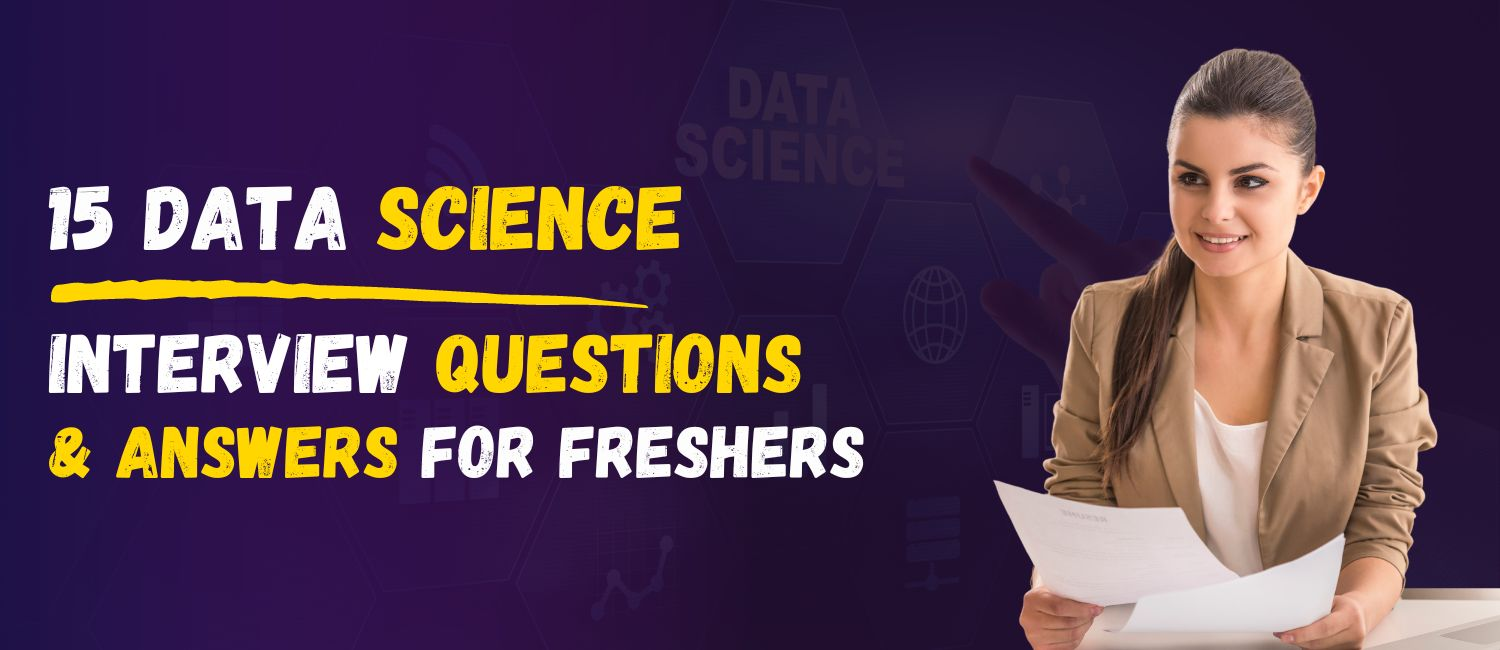15 Data Science Interview Questions and Answers for Fresher’s# 15 Data Science Interview Questions and Answers for Fresher’s

## Are you a student preparing for a Data Science Interview?

If so, you may be feeling overwhelmed by the amount of questions you need to prepare for. But don't worry! Our blog post today will provide you with 15 Data Science Interview Questions and Answers specifically tailored for Fresher’s.

We'll give you the insider knowledge you need to succeed in the interview, giving you the necessary confidence to ace the Data Science jobs. So keep reading to get the answers you need!

### Here is the List of 15 Data Science Interview Questions and Answers for Fresher’s

#### 1. What is Data Science?

Data science is a field that combines computer science, mathematics, and statistics to analyze large datasets and gain insights from them. It is used to solve complex problems and make predictions in various fields such as healthcare, finance, and marketing.

#### 2. What is the difference between supervised and unsupervised learning?

Supervised learning is a type of machine learning where the model is trained using labeled datasets. The model learns to make predictions by correlating the input data with the labeled data. Unsupervised learning is a type of machine learning where the model is trained using unlabeled datasets. The model learns to make predictions by detecting patterns in the data.

#### 3. What is the difference between a data scientist and a data analyst?

A data scientist is someone who is well-versed in both technical and analytical skills. They are able to analyze large datasets and develop predictive models to solve complex problems. A data analyst is someone who uses data to gain insights and make decisions. They are not as technical as data scientists and they do not develop predictive models.

#### 4. What is the difference between linear regression and logistic regression?

Linear regression is a type of predictive model used to identify linear relationships between a dependent variable and one or more independent variables. Logistic regression is a type of predictive model used to identify the probability of a binary outcome.

#### 5. What is an algorithm?

An algorithm is a set of instructions or steps used to solve a problem or complete a task. It is a step-by-step process used to achieve a desired result.

#### 6. What is the difference between a decision tree and a random forest?

A decision tree is a type of predictive model used to identify the best decision based on a set of conditions. A random forest is a type of predictive model that uses multiple decision trees to make predictions.

#### 7. What is the K-means clustering algorithm?

K-means clustering is an unsupervised machine learning algorithm used for clustering data points into ‘k’ clusters. The algorithm works by finding the centroid of each cluster and then assigning the data points to the closest centroid.

#### 8. What is a neural network?

A neural network is a type of machine learning algorithm that is modeled after the human brain. It consists of multiple layers of interconnected nodes that are used to detect patterns and make predictions.

#### 9. What is the difference between a false positive and a false negative?

A false positive is when a predictive model incorrectly predicts a positive result. A false negative is when a predictive model incorrectly predicts a negative result.

#### 10. What is the difference between a random variable and a parameter?

A random variable is a variable that takes on different values based on a set of conditions. A parameter is an estimate of the value of a random variable.

#### 11. What is the difference between a decision tree and a neural network?

A decision tree is a type of predictive model used to identify the best decision based on a set of conditions. A neural network is a type of machine learning algorithm that is modeled after the human brain.

#### 12. What is the difference between a supervised and unsupervised learning algorithm?

Supervised learning algorithms are trained using labeled datasets. The model learns to make predictions by correlating the input data with the labeled data. Unsupervised learning algorithms are trained using unlabeled datasets. The model learns to make predictions by detecting patterns in the data.

#### 13. What is the difference between a regression model and a classification model?

A regression model is a type of predictive model used to identify linear relationships between a dependent variable and one or more independent variables. A classification model is a type of predictive model used to identify the probability of a binary outcome.

#### 14. What is the Naive Bayes algorithm?

The Naive Bayes algorithm is a type of machine learning algorithm used to classify data points into different categories. It works by calculating the probability of a given outcome based on the prior probabilities of each category.

#### 15. What is the difference between a supervised and unsupervised learning algorithm?

Supervised learning algorithms are trained using labeled datasets. The model learns to make predictions by correlating the input data with the labeled data. Unsupervised learning algorithms are trained using unlabeled datasets. The model learns to make predictions by detecting patterns in the data.

#### Conclusion

Data Science is an ever-evolving field, and interview preparation is essential to ensure that you stand out from the crowd. The 15 Data Science Interview Questions and Answers for Fresher’s outlined in this article provide insight into the types of questions you are likely to encounter during your interview. If you want to learn Data Science Course, join ThinkNEXT for Data Science Course to make your career. While no single answer is guaranteed to guarantee success in your interview, familiarizing yourself with these questions and their potential answers can go a long way in helping you to make a good impression on the interviewer.